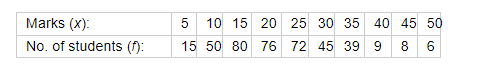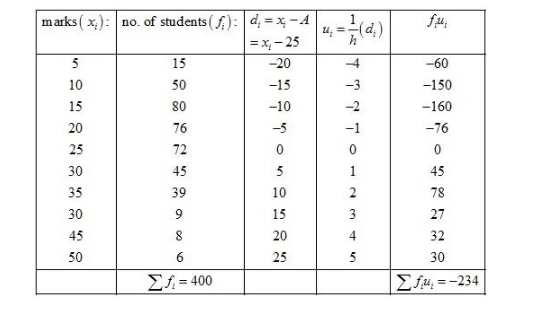# Find the mean from the following frequency distribution of marks at a test in statistics:

Question:

Find the mean from the following frequency distribution of marks at a test in statistics:Solution:

Let the assumed mean be $A=25$ and $h=5$.We know that mean, $\bar{X}=A+h\left(\frac{1}{N} \sum_{i=1}^{n} f_{i} u_{i}\right)$

Now, we have $N=\sum f_{i}=400, \sum f_{i} u_{i}=-234, h=5$ and $A=25$.

Putting the values in the above formula, we get

$\bar{X}=A+h\left(\frac{1}{N} \sum_{i=1}^{n} f_{i} u_{i}\right)$

$=25+5\left(\frac{1}{400} \times(-234)\right)$

$=25-\frac{234}{80}$

$=25-2.925$

$=22.075$

Hence, the mean marks is 22.075.